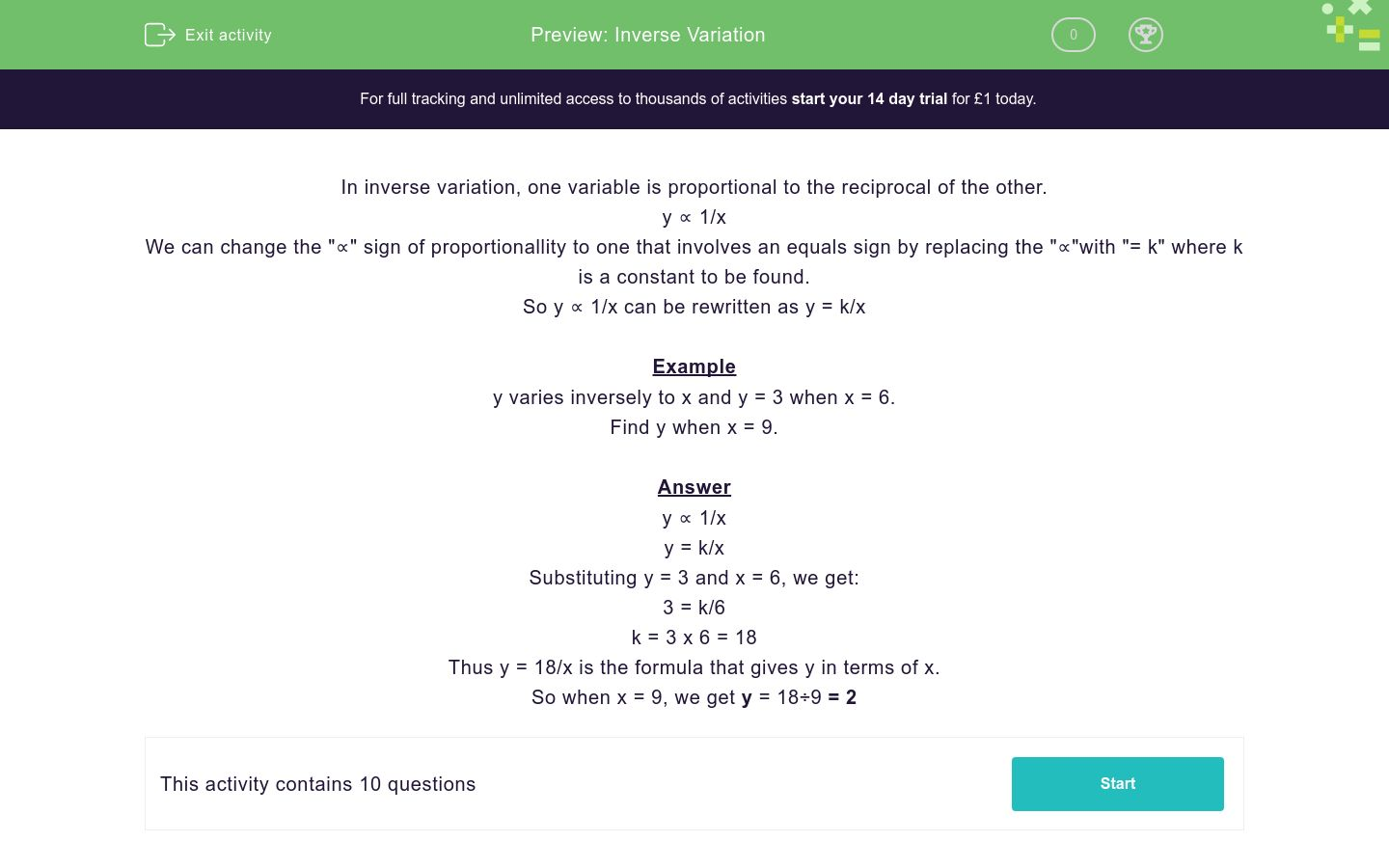# Inverse Variation

In this worksheet, students use constants to work out inverse variations.Key stage:  KS 4

Curriculum topic:  Ratio, Proportion and Rates of Change

Curriculum subtopic:  Interpret Equations that Describe Direct and Inverse Proportion

Difficulty level:### QUESTION 1 of 10

In inverse variation, one variable is proportional to the reciprocal of the other.

y ∝ 1/x

We can change the "∝" sign of proportionallity to one that involves an equals sign by replacing the "∝"with "= k" where k is a constant to be found.

So y ∝ 1/x can be rewritten as y = k/x

Example

y varies inversely to x and y = 3 when x = 6.

Find y when x = 9.

y ∝ 1/x

y = k/x

Substituting y = 3 and x = 6, we get:

3 = k/6

k = 3 x 6 = 18

Thus y = 18/x is the formula that gives y in terms of x.

So when x = 9, we get y = 18÷9 = 2

y varies inversely to x and y = 3 when x = 9.

By first writing y ∝ 1/x and then changing this to y = k/x, find the value of the constant k.

y varies inversely to x and y = 3 when x = 9.

Find y when x = 3.

y varies inversely to x and y = 3 when x = 8.

By first writing y ∝ 1/x and then changing this to y = k/x, find the value of the constant k.

y varies inversely to x and y = 3 when x = 8.

Find y when x = 6.

y varies inversely to x and y = 3 when x = 3.

By first writing y ∝ 1/x and then changing this to y = k/x, find the value of the constant k.

y varies inversely to x and y = 3 when x = 3.

Find y when x = 1.

y varies inversely to x and y = 2 when x = 12.

By first writing y ∝ 1/x and then changing this to y = k/x, find the value of the constant k.

y varies inversely to x and y = 2 when x = 12.

Find x when y = 3.

y varies inversely to x and y = 3 when x = 12.

By first writing y ∝ 1/x and then changing this to y = k/x, find the value of the constant k.

y varies inversely to x and y = 3 when x = 12.

Find x when y = 18.

• Question 1

y varies inversely to x and y = 3 when x = 9.

By first writing y ∝ 1/x and then changing this to y = k/x, find the value of the constant k.

27
EDDIE SAYS
3 = k/9
• Question 2

y varies inversely to x and y = 3 when x = 9.

Find y when x = 3.

9
EDDIE SAYS
3 = k/9
k = 27
y = 27/x
y = 27/3
• Question 3

y varies inversely to x and y = 3 when x = 8.

By first writing y ∝ 1/x and then changing this to y = k/x, find the value of the constant k.

24
EDDIE SAYS
3 = k/8
• Question 4

y varies inversely to x and y = 3 when x = 8.

Find y when x = 6.

4
EDDIE SAYS
3 = k/8
k = 24
y = 24/x
y = 24/6
• Question 5

y varies inversely to x and y = 3 when x = 3.

By first writing y ∝ 1/x and then changing this to y = k/x, find the value of the constant k.

9
EDDIE SAYS
3 = k/3
• Question 6

y varies inversely to x and y = 3 when x = 3.

Find y when x = 1.

9
EDDIE SAYS
3 = k/3
k = 9
y = 9/x
y = 9/1
• Question 7

y varies inversely to x and y = 2 when x = 12.

By first writing y ∝ 1/x and then changing this to y = k/x, find the value of the constant k.

24
EDDIE SAYS
2 = k/12
• Question 8

y varies inversely to x and y = 2 when x = 12.

Find x when y = 3.

8
EDDIE SAYS
2 = k/12
k = 24
y = 24/x
3 = 24/x
• Question 9

y varies inversely to x and y = 3 when x = 12.

By first writing y ∝ 1/x and then changing this to y = k/x, find the value of the constant k.

36
EDDIE SAYS
3 = k/12
• Question 10

y varies inversely to x and y = 3 when x = 12.

Find x when y = 18.

2
EDDIE SAYS
3 = k/12
k = 36
y = 36/x
18 = 36/x
---- OR ----

Sign up for a £1 trial so you can track and measure your child's progress on this activity.

### What is EdPlace?

We're your National Curriculum aligned online education content provider helping each child succeed in English, maths and science from year 1 to GCSE. With an EdPlace account you’ll be able to track and measure progress, helping each child achieve their best. We build confidence and attainment by personalising each child’s learning at a level that suits them.